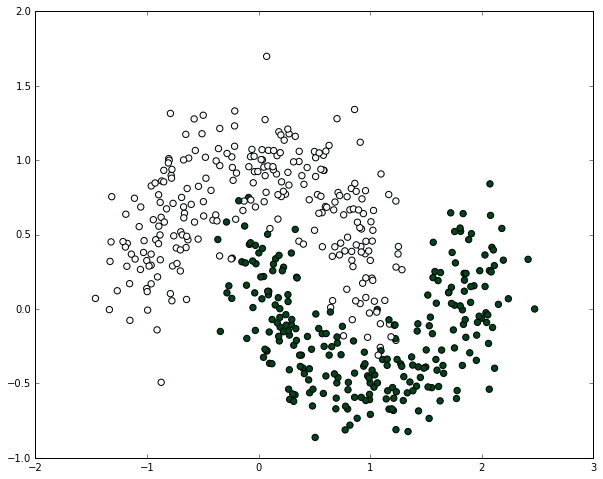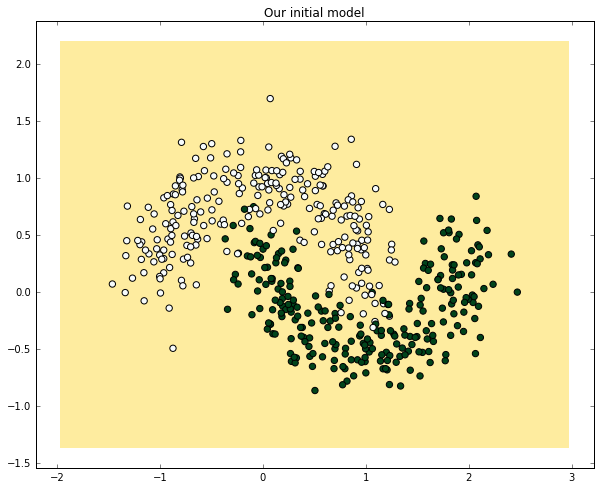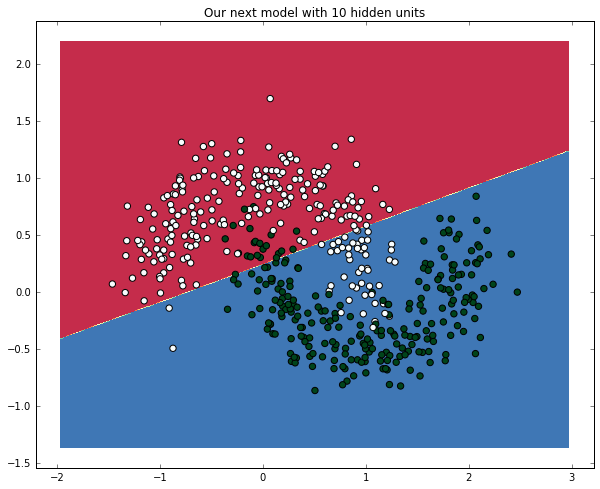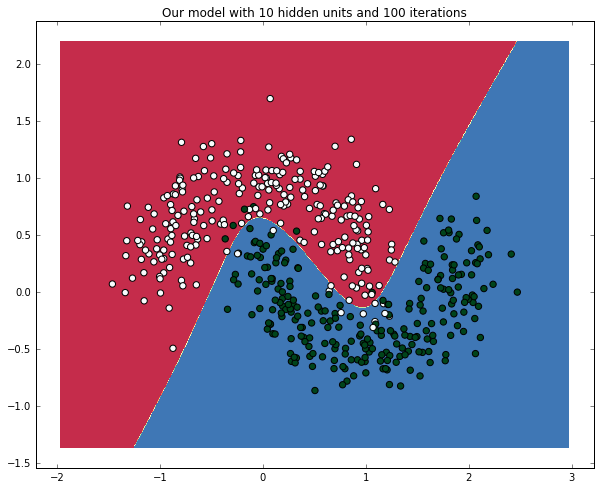Credits: Forked from deep-learning-keras-tensorflow by Valerio Maggio

# Introduction to Deep Learning¶

Deep learning allows computational models that are composed of multiple processing layers to learn representations of data with multiple levels of abstraction.

These methods have dramatically improved the state-of-the-art in speech recognition, visual object recognition, object detection and many other domains such as drug discovery and genomics.

Deep learning is one of the leading tools in data analysis these days and one of the most common frameworks for deep learning is Keras.

The Tutorial will provide an introduction to deep learning using keras with practical code examples.

# Artificial Neural Networks (ANN)¶

In machine learning and cognitive science, an artificial neural network (ANN) is a network inspired by biological neural networks which are used to estimate or approximate functions that can depend on a large number of inputs that are generally unknown

An ANN is built from nodes (neurons) stacked in layers between the feature vector and the target vector.

A node in a neural network is built from Weights and Activation function

An early version of ANN built from one node was called the PerceptronThe Perceptron is an algorithm for supervised learning of binary classifiers. functions that can decide whether an input (represented by a vector of numbers) belongs to one class or another.

Much like logistic regression, the weights in a neural net are being multiplied by the input vertor summed up and feeded into the activation function's input.

A Perceptron Network can be designed to have multiple layers, leading to the Multi-Layer Perceptron (aka MLP)The weights of each neuron are learned by gradient descent, where each neuron's error is derived with respect to it's weight.

Optimization is done for each layer with respect to the previous layer in a technique known as BackPropagation.# Building Neural Nets from scratch¶

### Idea:¶

We will build the neural networks from first principles. We will create a very simple model and understand how it works. We will also be implementing backpropagation algorithm.

Please note that this code is not optimized and not to be used in production.

This is for instructive purpose - for us to understand how ANN works.

Libraries like theano have highly optimized code.

(The following code is inspired from these terrific notebooks)

In :
# Import the required packages
import numpy as np
import pandas as pd
import matplotlib
import matplotlib.pyplot as plt
import scipy

In :
# Display plots inline
%matplotlib inline
# Define plot's default figure size
matplotlib.rcParams['figure.figsize'] = (10.0, 8.0)

In :
import random
random.seed(123)

In :
#read the datasets

In :
X, y = np.array(train.ix[:,0:2]), np.array(train.ix[:,2])

In :
X.shape

Out:
(500, 2)
In :
y.shape

Out:
(500,)
In :
#Let's plot the dataset and see how it is
plt.scatter(X[:,0], X[:,1], s=40, c=y, cmap=plt.cm.BuGn)

Out:
<matplotlib.collections.PathCollection at 0x110b4b0f0>## Start Building our ANN building blocks¶

Note: This process will eventually result in our own Neural Networks class

### A look at the details¶### Function to generate a random number, given two numbers¶

Where will it be used?: When we initialize the neural networks, the weights have to be randomly assigned.

In :
# calculate a random number where:  a <= rand < b
def rand(a, b):
return (b-a)*random.random() + a

In :
# Make a matrix
def makeMatrix(I, J, fill=0.0):
return np.zeros([I,J])


### Define our activation function. Let's use sigmoid function¶

In :
# our sigmoid function
def sigmoid(x):
#return math.tanh(x)
return 1/(1+np.exp(-x))


### Derivative of our activation function.¶

Note: We need this when we run the backpropagation algorithm

In :
# derivative of our sigmoid function, in terms of the output (i.e. y)
def dsigmoid(y):
return y - y**2


### Our neural networks class¶

When we first create a neural networks architecture, we need to know the number of inputs, number of hidden layers and number of outputs.

The weights have to be randomly initialized.

class ANN:
def __init__(self, ni, nh, no):
# number of input, hidden, and output nodes
self.ni = ni + 1 # +1 for bias node
self.nh = nh
self.no = no

# activations for nodes
self.ai = [1.0]*self.ni
self.ah = [1.0]*self.nh
self.ao = [1.0]*self.no

# create weights
self.wi = makeMatrix(self.ni, self.nh)
self.wo = makeMatrix(self.nh, self.no)

# set them to random vaules
self.wi = rand(-0.2, 0.2, size=self.wi.shape)
self.wo = rand(-2.0, 2.0, size=self.wo.shape)

# last change in weights for momentum
self.ci = makeMatrix(self.ni, self.nh)
self.co = makeMatrix(self.nh, self.no)


### Activation Function¶

def activate(self, inputs):

if len(inputs) != self.ni-1:
print(inputs)
raise ValueError('wrong number of inputs')

# input activations
for i in range(self.ni-1):
self.ai[i] = inputs[i]

# hidden activations
for j in range(self.nh):
sum_h = 0.0
for i in range(self.ni):
sum_h += self.ai[i] * self.wi[i][j]
self.ah[j] = sigmoid(sum_h)

# output activations
for k in range(self.no):
sum_o = 0.0
for j in range(self.nh):
sum_o += self.ah[j] * self.wo[j][k]
self.ao[k] = sigmoid(sum_o)

return self.ao[:]


### BackPropagation¶

def backPropagate(self, targets, N, M):

if len(targets) != self.no:
print(targets)
raise ValueError('wrong number of target values')

# calculate error terms for output
output_deltas = np.zeros(self.no)
for k in range(self.no):
error = targets[k]-self.ao[k]
output_deltas[k] = dsigmoid(self.ao[k]) * error

# calculate error terms for hidden
hidden_deltas = np.zeros(self.nh)
for j in range(self.nh):
error = 0.0
for k in range(self.no):
error += output_deltas[k]*self.wo[j][k]
hidden_deltas[j] = dsigmoid(self.ah[j]) * error

# update output weights
for j in range(self.nh):
for k in range(self.no):
change = output_deltas[k] * self.ah[j]
self.wo[j][k] += N*change +
M*self.co[j][k]
self.co[j][k] = change

# update input weights
for i in range(self.ni):
for j in range(self.nh):
change = hidden_deltas[j]*self.ai[i]
self.wi[i][j] += N*change +
M*self.ci[i][j]
self.ci[i][j] = change

# calculate error
error = 0.0
for k in range(len(targets)):
error += 0.5*(targets[k]-self.ao[k])**2
return error

In :
# Putting all together

class ANN:
def __init__(self, ni, nh, no):
# number of input, hidden, and output nodes
self.ni = ni + 1 # +1 for bias node
self.nh = nh
self.no = no

# activations for nodes
self.ai = [1.0]*self.ni
self.ah = [1.0]*self.nh
self.ao = [1.0]*self.no

# create weights
self.wi = makeMatrix(self.ni, self.nh)
self.wo = makeMatrix(self.nh, self.no)

# set them to random vaules
for i in range(self.ni):
for j in range(self.nh):
self.wi[i][j] = rand(-0.2, 0.2)
for j in range(self.nh):
for k in range(self.no):
self.wo[j][k] = rand(-2.0, 2.0)

# last change in weights for momentum
self.ci = makeMatrix(self.ni, self.nh)
self.co = makeMatrix(self.nh, self.no)

def backPropagate(self, targets, N, M):

if len(targets) != self.no:
print(targets)
raise ValueError('wrong number of target values')

# calculate error terms for output
output_deltas = np.zeros(self.no)
for k in range(self.no):
error = targets[k]-self.ao[k]
output_deltas[k] = dsigmoid(self.ao[k]) * error

# calculate error terms for hidden
hidden_deltas = np.zeros(self.nh)
for j in range(self.nh):
error = 0.0
for k in range(self.no):
error += output_deltas[k]*self.wo[j][k]
hidden_deltas[j] = dsigmoid(self.ah[j]) * error

# update output weights
for j in range(self.nh):
for k in range(self.no):
change = output_deltas[k] * self.ah[j]
self.wo[j][k] += N*change + M*self.co[j][k]
self.co[j][k] = change

# update input weights
for i in range(self.ni):
for j in range(self.nh):
change = hidden_deltas[j]*self.ai[i]
self.wi[i][j] += N*change + M*self.ci[i][j]
self.ci[i][j] = change

# calculate error
error = 0.0
for k in range(len(targets)):
error += 0.5*(targets[k]-self.ao[k])**2
return error

def test(self, patterns):
self.predict = np.empty([len(patterns), self.no])
for i, p in enumerate(patterns):
self.predict[i] = self.activate(p)
#self.predict[i] = self.activate(p)

def activate(self, inputs):

if len(inputs) != self.ni-1:
print(inputs)
raise ValueError('wrong number of inputs')

# input activations
for i in range(self.ni-1):
self.ai[i] = inputs[i]

# hidden activations
for j in range(self.nh):
sum_h = 0.0
for i in range(self.ni):
sum_h += self.ai[i] * self.wi[i][j]
self.ah[j] = sigmoid(sum_h)

# output activations
for k in range(self.no):
sum_o = 0.0
for j in range(self.nh):
sum_o += self.ah[j] * self.wo[j][k]
self.ao[k] = sigmoid(sum_o)

return self.ao[:]

def train(self, patterns, iterations=1000, N=0.5, M=0.1):
# N: learning rate
# M: momentum factor
patterns = list(patterns)
for i in range(iterations):
error = 0.0
for p in patterns:
inputs = p
targets = p
self.activate(inputs)
error += self.backPropagate([targets], N, M)
if i % 5 == 0:
print('error in interation %d : %-.5f' % (i,error))
print('Final training error: %-.5f' % error)


### Running the model on our dataset¶

In :
# create a network with two inputs, one hidden, and one output nodes
ann = ANN(2, 1, 1)

%timeit -n 1 -r 1 ann.train(zip(X,y), iterations=2)

error in interation 0 : 53.62995
Final training error: 53.62995
Final training error: 47.35136
1 loop, best of 1: 97.6 ms per loop


### Predicting on training dataset and measuring in-sample accuracy¶

In :
%timeit -n 1 -r 1 ann.test(X)

1 loop, best of 1: 22.6 ms per loop

In :
prediction = pd.DataFrame(data=np.array([y, np.ravel(ann.predict)]).T,
columns=["actual", "prediction"])

Out:
actual prediction
0 1.0 0.491100
1 1.0 0.495469
2 0.0 0.097362
3 0.0 0.400006
4 1.0 0.489664
In :
np.min(prediction.prediction)

Out:
0.076553078113180129

### Let's visualize and observe the results¶

In :
# Helper function to plot a decision boundary.
# This generates the contour plot to show the decision boundary visually
def plot_decision_boundary(nn_model):
# Set min and max values and give it some padding
x_min, x_max = X[:, 0].min() - .5, X[:, 0].max() + .5
y_min, y_max = X[:, 1].min() - .5, X[:, 1].max() + .5
h = 0.01
# Generate a grid of points with distance h between them
xx, yy = np.meshgrid(np.arange(x_min, x_max, h),
np.arange(y_min, y_max, h))
# Predict the function value for the whole gid
nn_model.test(np.c_[xx.ravel(), yy.ravel()])
Z = nn_model.predict
Z[Z>=0.5] = 1
Z[Z<0.5] = 0
Z = Z.reshape(xx.shape)
# Plot the contour and training examples
plt.contourf(xx, yy, Z, cmap=plt.cm.Spectral)
plt.scatter(X[:, 0], X[:, 1], s=40,  c=y, cmap=plt.cm.BuGn)

In :
plot_decision_boundary(ann)
plt.title("Our initial model")

Out:
<matplotlib.text.Text at 0x110bdb940>Exercise:

Create Neural networks with 10 hidden nodes on the above code.

What's the impact on accuracy?

In :
# Put your code here
#(or load the solution if you wanna cheat :-)

In :
# %load solutions/sol_111.py
ann = ANN(2, 10, 1)
%timeit -n 1 -r 1 ann.train(zip(X,y), iterations=2)
plot_decision_boundary(ann)
plt.title("Our next model with 10 hidden units")

error in interation 0 : 34.91394
Final training error: 34.91394
Final training error: 25.36183
1 loop, best of 1: 288 ms per loop

Out:
<matplotlib.text.Text at 0x11151f630>Exercise:

Train the neural networks by increasing the epochs.

What's the impact on accuracy?

In :
#Put your code here

In :
# %load solutions/sol_112.py
ann = ANN(2, 10, 1)
%timeit -n 1 -r 1 ann.train(zip(X,y), iterations=100)
plot_decision_boundary(ann)
plt.title("Our model with 10 hidden units and 100 iterations")

error in interation 0 : 31.63185
Final training error: 31.63185
Final training error: 25.12319
Final training error: 24.92547
Final training error: 24.89692
Final training error: 24.88124
error in interation 5 : 24.86083
Final training error: 24.86083
Final training error: 24.83512
Final training error: 24.80603
Final training error: 24.77544
Final training error: 24.74477
error in interation 10 : 24.71498
Final training error: 24.71498
Final training error: 24.68669
Final training error: 24.66021
Final training error: 24.63569
Final training error: 24.61313
error in interation 15 : 24.59246
Final training error: 24.59246
Final training error: 24.57359
Final training error: 24.55639
Final training error: 24.54072
Final training error: 24.52646
error in interation 20 : 24.51350
Final training error: 24.51350
Final training error: 24.50171
Final training error: 24.49100
Final training error: 24.48127
Final training error: 24.47242
error in interation 25 : 24.46436
Final training error: 24.46436
Final training error: 24.45702
Final training error: 24.45030
Final training error: 24.44414
Final training error: 24.43846
error in interation 30 : 24.43319
Final training error: 24.43319
Final training error: 24.42828
Final training error: 24.42366
Final training error: 24.41929
Final training error: 24.41510
error in interation 35 : 24.41107
Final training error: 24.41107
Final training error: 24.40715
Final training error: 24.40331
Final training error: 24.39952
Final training error: 24.39576
error in interation 40 : 24.39200
Final training error: 24.39200
Final training error: 24.38821
Final training error: 24.38438
Final training error: 24.38048
Final training error: 24.37649
error in interation 45 : 24.37237
Final training error: 24.37237
Final training error: 24.36806
Final training error: 24.36353
Final training error: 24.35868
Final training error: 24.35340
error in interation 50 : 24.34754
Final training error: 24.34754
Final training error: 24.34086
Final training error: 24.33302
Final training error: 24.32348
Final training error: 24.31138
error in interation 55 : 24.29529
Final training error: 24.29529
Final training error: 24.27275
Final training error: 24.23928
Final training error: 24.18646
Final training error: 24.09789
error in interation 60 : 23.94185
Final training error: 23.94185
Final training error: 23.66093
Final training error: 23.16905
Final training error: 22.38000
Final training error: 21.27360
error in interation 65 : 19.93871
Final training error: 19.93871
Final training error: 18.52756
Final training error: 17.16893
Final training error: 15.93090
Final training error: 14.83582
error in interation 70 : 13.88300
Final training error: 13.88300
Final training error: 13.06081
Final training error: 12.35255
Final training error: 11.74050
Final training error: 11.20877
error in interation 75 : 10.74440
Final training error: 10.74440
Final training error: 10.33728
Final training error: 9.97939
Final training error: 9.66423
Final training error: 9.38631
error in interation 80 : 9.14093
Final training error: 9.14093
Final training error: 8.92402
Final training error: 8.73205
Final training error: 8.56193
Final training error: 8.41096
error in interation 85 : 8.27675
Final training error: 8.27675
Final training error: 8.15722
Final training error: 8.05052
Final training error: 7.95506
Final training error: 7.86944
error in interation 90 : 7.79246
Final training error: 7.79246
Final training error: 7.72306
Final training error: 7.66035
Final training error: 7.60354
Final training error: 7.55195
error in interation 95 : 7.50499
Final training error: 7.50499
Final training error: 7.46215
Final training error: 7.42298
Final training error: 7.38707
Final training error: 7.35410
1 loop, best of 1: 14.5 s per loop

Out:
<matplotlib.text.Text at 0x1115951d0>